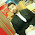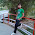## Solved Objective Questions from Induction Motors

 The crawling in an induction motor is caused by
(a) Improper design of the machine
(b) Low voltage supply
(d) Harmonics developed in the motor

 The speed of an induction motor

(a) Decreases too much with the increase of load
(b) Increase with the increase of load
(c) Decreases slightly with the increase of load
(d) Remains constant with the increase of load

 The effect of increasing the length of the air gap in an induction motor will increase

(a) Power factor
(b) Speed
(c) Magnetising current
(d) Air-gap flux

 the difference between the synchronous speed and the actual speed of an induction motor is known as
(a) Regulation
(b) Back lash
(c) Slip
(d) Lag

 Rotating magnetic field is produced in a....

(a) Single - phase induction motor
(b) Three phase induction motor
(c) DC series motor
(d) AC series motor

 The stator core of the induction motor is made of

(a) Laminated cast iron
(b) Mild steel
(c) Silicon steel stampings
(d) Soft wood

 Star- delta starter of an induction motor

(a) Inserts resistance in rotor circuit
(b) Inserts resistance in stator circuit
(c) Applies reduced voltage to rotor
(d) Applies reduced voltage to stator

 The starting torque of a 1-phase induction motor is

(a) High
(b) Medium
(c) Low
(d) Zero

 The thrust developed by a linear induction motor depends on

(a) Synchronous speed
(b) Rotor input
(c) Number of poles
(d) Both A and B

 A 3-phase squirrel cage induction motor supplied from a balanced 3-phase source drives a mechanical load. The torque-speed characteristics of the motor (solid curve) and of the load (dotted curve) are shown. Of the two equilibrium points A and B, which of the following options correctly describes the stability of A and B?
[GATE 2009](a) A is stable B is unstable
(b) A is unstable B is stable
(c) Both are stable
(d) Both are unstable
 If an induction machine is run at above synchronous speed, it acts as [Gate 1997]
(a) A synchronous motor
(b) An induction generator
(c) An induction motor
(d) None of the above

8:04 PM

1.2.Thank you

3.Thanks for giving such good questions * b

4.5.I guess answer of 10 is A